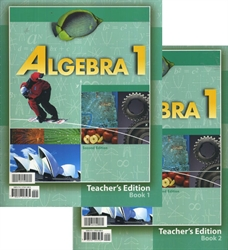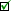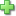# Algebra 1 - Teacher Edition (old)

Spiralbound, 640 pages
Current Retail Price: \$60.00
Used Price: \$8.00 (1 in stock)Condition Policy

BJU's Algebra 1 presents algebra topics in a logical order. The text develops an understanding of algebra by justifying methods and by explaining how to do the problems. It introduces graphing, solving systems of equations, operations with polynomials and radicals, factoring polynomials, solving rational equations, and graphing quadratic functions. Biographical sketches of mathematicians are included, as well as features on probability and statistics, Algebra Around Us, and Algebra and Scripture.

The Teacher Edition includes student pages with notes and answers (including solutions) and sections with common student errors and one-on-one activities.

Did you find this review helpful?
Related CategoriesClick here to write a review